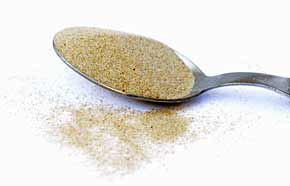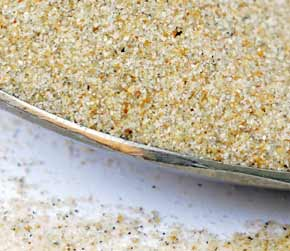﻿ Beach Sand 1 cubic centimeter volume to cubic millimeters converter

Category: main menubeach sand menuCubic centimeters

# beach sand conversion

## Amount: 1 cubic centimeter (cc - cm3) of volume Equals: 1,000.00 cubic millimeters (mm3) in volume

Converting cubic centimeter to cubic millimeters value in the beach sand units scale.

TOGGLE :   from cubic millimeters into cubic centimeters in the other way around.

## beach sand from cubic centimeter to cubic millimeter Conversion Results:

### Enter a New cubic centimeter Amount of beach sand to Convert From

* Whole numbers, decimals or fractions (ie: 6, 5.33, 17 3/8)
* Precision is how many numbers after decimal point (1 - 9)

Enter Amount :
Decimal Precision :

CONVERT :   between other beach sand measuring units - complete list.

Conversion calculator for webmasters.

## Beach sand weight vs. volume units

Beach sand has quite high density, it's heavy and it easily leaks into even tiny gaps or other opened spaces. No wonder it absorbs and conducts heat energy from the sun so well. However, this sand does not have the heat conductivity as high as glass does, or fireclay and firebricks, or dense concrete. A fine beach sand in dry form was used for taking these measurements.Convert beach sand measuring units between cubic centimeter (cc - cm3) and cubic millimeters (mm3) but in the other reverse direction from cubic millimeters into cubic centimeters.

 conversion result for beach sand: From Symbol Equals Result To Symbol 1 cubic centimeter cc - cm3 = 1,000.00 cubic millimeters mm3

# Converter type: beach sand measurements

This online beach sand from cc - cm3 into mm3 converter is a handy tool not just for certified or experienced professionals.

First unit: cubic centimeter (cc - cm3) is used for measuring volume.
Second: cubic millimeter (mm3) is unit of volume.

## beach sand per 1,000.00 mm3 is equivalent to 1 what?

The cubic millimeters amount 1,000.00 mm3 converts into 1 cc - cm3, one cubic centimeter. It is the EQUAL beach sand volume value of 1 cubic centimeter but in the cubic millimeters volume unit alternative.

How to convert 2 cubic centimeters (cc - cm3) of beach sand into cubic millimeters (mm3)? Is there a calculation formula?

First divide the two units variables. Then multiply the result by 2 - for example:
1000 * 2 (or divide it by / 0.5)

QUESTION:
1 cc - cm3 of beach sand = ? mm3

ANSWER:
1 cc - cm3 = 1,000.00 mm3 of beach sand

## Other applications for beach sand units calculator ...

With the above mentioned two-units calculating service it provides, this beach sand converter proved to be useful also as an online tool for:
1. practicing cubic centimeters and cubic millimeters of beach sand ( cc - cm3 vs. mm3 ) measuring values exchange.
2. beach sand amounts conversion factors - between numerous unit pairs variations.
3. working with mass density - how heavy is a volume of beach sand - values and properties.

International unit symbols for these two beach sand measurements are:

Abbreviation or prefix ( abbr. short brevis ), unit symbol, for cubic centimeter is:
cc - cm3
Abbreviation or prefix ( abbr. ) brevis - short unit symbol for cubic millimeter is:
mm3

### One cubic centimeter of beach sand converted to cubic millimeter equals to 1,000.00 mm3

How many cubic millimeters of beach sand are in 1 cubic centimeter? The answer is: The change of 1 cc - cm3 ( cubic centimeter ) volume unit of beach sand measure equals = to volume 1,000.00 mm3 ( cubic millimeter ) as the equivalent measure within the same beach sand substance type.

In principle with any measuring task, switched on professional people always ensure, and their success depends on, they get the most precise conversion results everywhere and every-time. Not only whenever possible, it's always so. Often having only a good idea ( or more ideas ) might not be perfect nor good enough solution. If there is an exact known measure in cc - cm3 - cubic centimeters for beach sand amount, the rule is that the cubic centimeter number gets converted into mm3 - cubic millimeters or any other beach sand unit absolutely exactly.

Conversion for how many cubic millimeters ( mm3 ) of beach sand are contained in a cubic centimeter ( 1 cc - cm3 ). Or, how much in cubic millimeters of beach sand is in 1 cubic centimeter? To link to this beach sand cubic centimeter to cubic millimeters online converter simply cut and paste the following.
The link to this tool will appear as: beach sand from cubic centimeter (cc - cm3) to cubic millimeters (mm3) conversion.

I've done my best to build this site for you- Please send feedback to let me know how you enjoyed visiting.